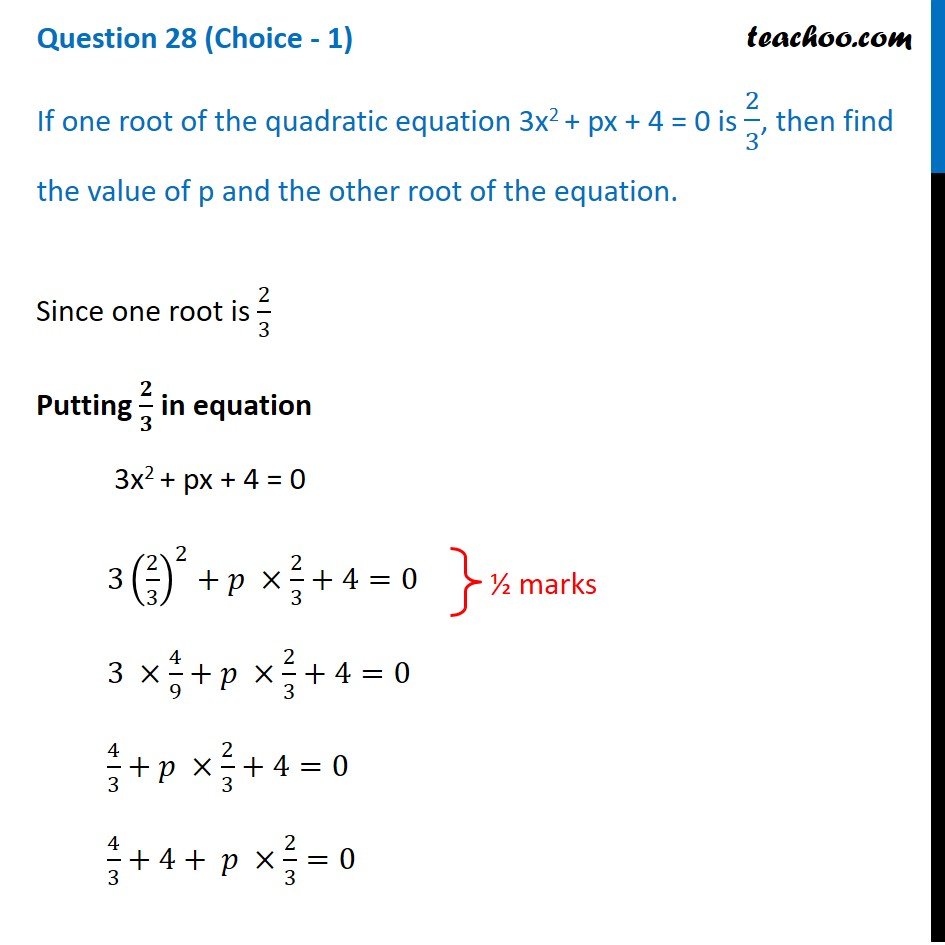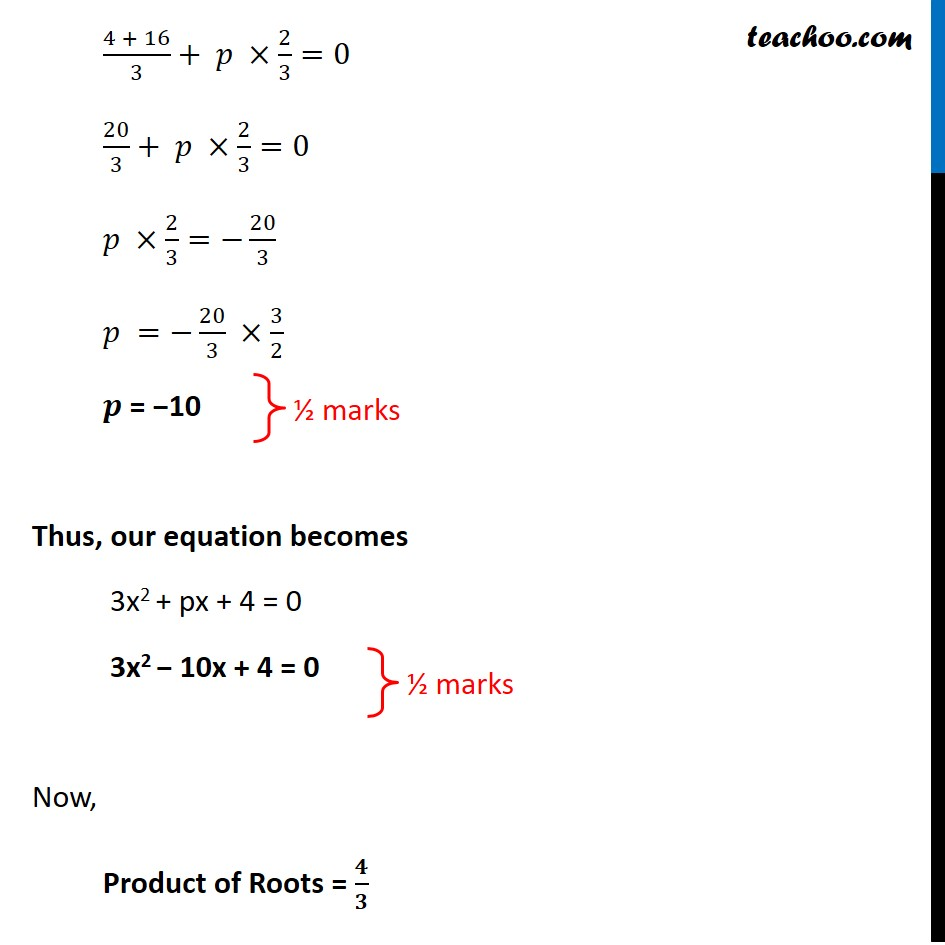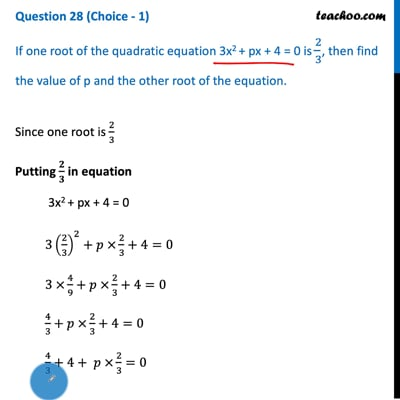CBSE Class 10 Sample Paper for 2021 Boards - Maths Standard

Class 10
Solutions of Sample Papers for Class 10 Boards

## If one root of the quadratic equation 3x 2 + px + 4 = 0 is 2/3, then find the value of p and the other root of the equation.This video is only available for Teachoo black users

Introducing your new favourite teacher - Teachoo Black, at only ₹83 per month

### Transcript

Question 28 (Choice - 1) If one root of the quadratic equation 3x2 + px + 4 = 0 is 2/3, then find the value of p and the other root of the equation.Since one root is 2/3 Putting 𝟐/𝟑 in equation 3x2 + px + 4 = 0 3(2/3)^2+𝑝 ×2/3+4=0 3 ×4/9+𝑝 ×2/3+4=0 4/3+𝑝 ×2/3+4=0 4/3+4+ 𝑝 ×2/3=0 (4 + 16)/3+ 𝑝 ×2/3=0 20/3+ 𝑝 ×2/3=0 𝑝 ×2/3=−20/3 𝑝 =−20/3 ×3/2 𝒑 = −10 Thus, our equation becomes 3x2 + px + 4 = 0 3x2 − 10x + 4 = 0 Now, Product of Roots = 𝟒/𝟑 First Root × Second Root = 4/3 2/3 × Second Root = 4/3 Second Root = 4/3 ×3/2 Second Root = 2